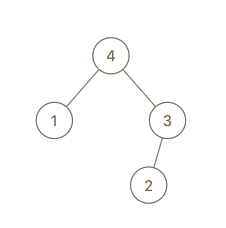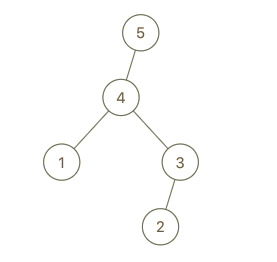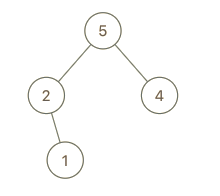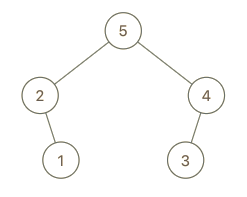# Monthly Archives: May 2019

## lc 654 Maximum Binary Tree

We are given the `root` node of a maximum tree: a tree where every node has a value greater than any other value in its subtree.

Just as in the previous problem, the given tree was constructed from an list `A` (`root = Construct(A)`) recursively with the following `Construct(A)` routine:

• If `A` is empty, return `null`.
• Otherwise, let `A[i]` be the largest element of `A`.  Create a `root` node with value `A[i]`.
• The left child of `root` will be `Construct([A, A, ..., A[i-1]])`
• The right child of `root` will be `Construct([A[i+1], A[i+2], ..., A[A.length - 1]])`
• Return `root`.

Note that we were not given A directly, only a root node `root = Construct(A)`.

Suppose `B` is a copy of `A` with the value `val` appended to it.  It is guaranteed that `B` has unique values.

Return `Construct(B)`.

Example 1:```Input: root = [4,1,3,null,null,2], val = 5
Output: [5,4,null,1,3,null,null,2]
Explanation: A = [1,4,2,3], B = [1,4,2,3,5]
```

Example 2:```Input: root = [5,2,4,null,1], val = 3
Output: [5,2,4,null,1,null,3]
Explanation: A = [2,1,5,4], B = [2,1,5,4,3]
```

Example 3:```Input: root = [5,2,3,null,1], val = 4
Output: [5,2,4,null,1,3]
Explanation: A = [2,1,5,3], B = [2,1,5,3,4]
```

Solution1: divide-and-conquer O(nlogn)

```public class Solution {
public TreeNode constructMaximumBinaryTree(int[] nums) {
if (nums == null) return null;
return build(nums, 0, nums.length - 1);
}

private TreeNode build(int[] nums, int start, int end) {
if (start > end) return null;

int idxMax = start;
for (int i = start + 1; i <= end; i++) {
if (nums[i] > nums[idxMax]) {
idxMax = i;
}
}

TreeNode root = new TreeNode(nums[idxMax]);

root.left = build(nums, start, idxMax - 1);
root.right = build(nums, idxMax + 1, end);

return root;
}
}```

Solution 2. Stack iteration, O(n)

At most each element comes and leave stack together. So at most, it takes 2N time.

Only the “right straight line” is in stack.For each iteration, the goal is to put curr somewhere in the “right straight line”.` `
```public static TreeNode constructMaximumBinaryTree(int[] nums) {
Stack<TreeNode> stack = new Stack<>();
for (int curr : nums) {
TreeNode node = new TreeNode(curr);
while (!stack.isEmpty() && stack.peek().val < curr) {
node.left = stack.pop();
}
if (!stack.isEmpty()) {
stack.peek().right = node;
}
stack.push(node);
}
return stack.get(0);
}```